# Role of Dispersive and Nonlinear Effects

So far we have considered amplifier noise without paying attention to how this noise interacts with the dispersive and nonlinear effects that also occur as an optical signal propagates along the fiber link. In reality, ASE noise propagates with the signal and is affected by the same dispersive and nonlinear mechanisms that affect the optical signal. This tutorial shows that ASE noise can be enhanced considerably if the conditions are suitable for modulation instability to occur. Moreover, ASE noise affects optical pulses and induces not only energy fluctuations but also timing jitter.

#### 1. Noise Growth through Modulation Instability

Consider a long-haul lightwave system with lumped amplifiers placed periodically along the link. Each amplifier adds ASE noise that propagates with the signal in multiple fiber sections. In a purely linear system, the noise power would not change. However, the nonlinear term in the NLS equation couples the ASE and signal and modifies both of them through the three nonlinear effects, SPM, XPM, and FWDM, discussed in this tutorial. In particular, the phenomenon of modulation instability degrades the system performance by amplifying the ASE noise and converting phase fluctuations into intensity noise.

To study how ASE noise is affected by modulation instability, we solve this NLS equation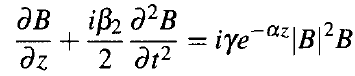assuming that a CW signal with power P0 enters the fiber with noise superimposed on it. Writing the solution in the form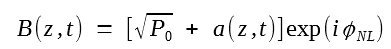where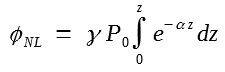is the SPM-induced nonlinear phase shift, and assuming that noise is much weaker than the signal (|a|2 ≪ P0), we obtainThis linear equation is earlier to solve in the Fourier domain because it reduces to the following set of two ordinary differential equations:where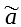represents the Fourier transform of a, and Ω = ωn - ω0 represents the shift of the noise frequency ωn from the signal carrier frequency ω0. These equations show that SPM couples the spectral components of ASE noise located symmetrically around ω0. When Ω falls within the gain bandwidth of modulation instability, the corresponding noise components are amplified. In the FWM picture, two photons of energy ћω0 from the signal are converted into two new photons with energies ћ(ω0 + Ω) and ћ(ω0 - Ω).

The coupled equations above can be solved easily when fiber losses are negligible (α = 0) so that the last term is z-independent. They can also be solved when α ≠ 0, but the solution involves the Hankel functions or modified Bessel functions of complex order and argument. A matrix method is also used for solving these equations numerically. To calculate the buildup of ASE noise in a long chain of amplifiers, one must add additional noise after each amplifier, propagate it in subsequent fiber sections, and sum the contribution for all amplifiers. This procedure allows one to calculate the optical spectrum as well as the relative intensity noise (RIN) spectrum at the receiver end. The following figure shows an example of a numerically simulated optical spectrum at the end of a 2,500-km fiber link with 50 amplifiers placed 50-km apart. A 1-mW signal at the 1.55-μm wavelength is transmitted through a fiber link using parameter values of β2 = -1 ps2/km, γ = 2 W-1/km, and α = 0.22 dB/km. Optical filters with 8-nm bandwidth filter the ASE after every amplifier. The broad pedestal represents the ASE spectrum expected even in the absence of nonlinear effects. The double-peak structure near the signal wavelength is due to modulation instability. The weak satellite peaks result from the nonlinear index grating formed by periodic variations of signal power along the fiber link.

We next focus on the degradation of optical signal as it propagates through a fiber link in which ASE noise is added by amplifiers periodically. For this purpose, we consider a single pulse representing bit 1 in a fixed time slot and ask how its energy and position are affected by noise. Although changes in pulse energy are expected, ASE can also induce timing jitter in a bit stream by shifting optical pulses from their original location within the time slot in a random fashion. Such a jitter was first studied in 1986 in the context of solitons and is called the Gordon-Haus jitter. It was recognized later that timing jitter occurs in any lightwave system employing optical amplifiers and it imposes a fundamental limitation on all long-haul systems.

The physical origin of ASE-induced jitter can be understood by noting that optical amplifiers affect not only the amplitude but also the phase of the amplified signal. Time-dependent variations in the optical phase shift the signal frequency from its original value ω0 by a small amount after each amplifier. Since the group velocity of an optical pulse depends on its carrier frequency (because of dispersion), the speed at which a pulse propagates through the fiber is affected by each amplifier in a random fashion. Such speed changes produce random shifts in the pulse position at the receiver and are responsible for the ASE-induced timing jitter.

In general, one should solve this following equation numerically to study how much signal is affected by noise.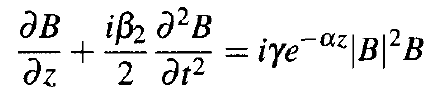However, such a Monte-Carlo approach is quite cumbersome in practice as this equation should be solved multiple times to collect a large enough statistical sample. The moment method allows one to perform the average over ASE noise analytically. It makes use of three moments E, q, and Ω, representing pulse energy, pulse-center position within the bit slot, and shift in the carrier frequency, respectively, and defined as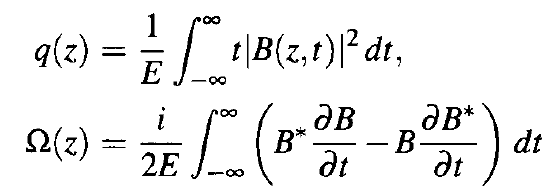whereAs the loss term has been removed from the first equation, we expect E to remain constant along the fiber in the absence of ASE noise.

Differentiating E, q, and Ω with respect to z, we find that they change in each passive fiber section asA simple integration shows that while pulse energy E and frequency Ω do not change during propagation, pulse position shifts if the pulse enters the fiber with a finite value of Ω. This is easily understood if we recall that the first equation is written in a frame moving with the group velocity vg of the pulse, that is, the center of the pulse remains stationary as long as the carrier frequency ω0 remains fixed. However, if this frequency shifts by Ω, the group velocity changes, and the pulse center shifts by q(z) = β2Ωz.

Consider now what happens when pulse enters the kth amplifier along the fiber link. Because of ASE added by the amplifier, E, Ω, and q change by random amounts δEk, δΩk, and δqk, respectively. It we include these random changes, we then get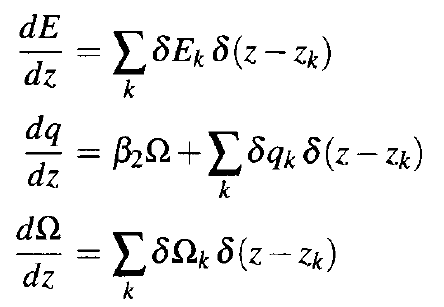where the sum is over the total number of amplifiers encountered by the pulse before it arrives at z. These equations show that frequency fluctuations induced by amplifiers manifest as position fluctuations because of GVD; some jitter occurs even when β2 = 0. Clearly, ASE-induced timing jitter would be large for fibers with large dispersion and can be reduced by operating a lightwave system near the zero-dispersion wavelength of the fiber.

We next consider the statistics of fluctuations δEk, δΩk, and δqk. Let B(zk, t) be the field entering the kth amplifier. After amplification, this field can be written as B(zk, t) + bk(t), where bk(t) is the ASE-induced change in the optical field. This change vanishes on average but its correlation function is given by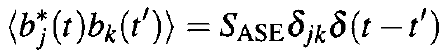where SASE is the spectral density of ASE and δjk indicates that fluctuations at two different amplifiers are not correlated. We can calculate the change in E, q, and Ω occurring after the kth amplifier. After some simplification, we obtain the following relations for these changes: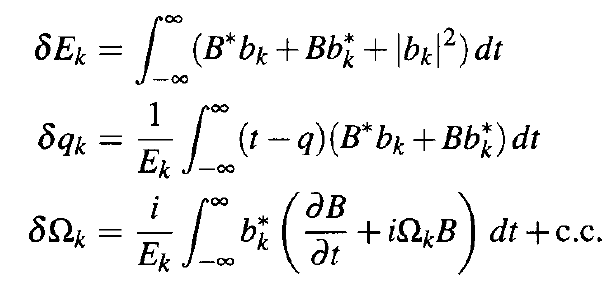We can now perform the average over the random variable bk together with ⟨bk⟩ = 0. Random variables δqk and δΩk vanish on average, but ⟨δEk⟩ = SASE. Their correlation and variances can also be calculated and are found to bewhere V = Bexp[iΩk(t - qk)]. These equations apply for any pulse shape. However, they require a knowledge of the optical field B(zk, t) at each amplifier. In the most general case, this field can be obtained by solving the first NLS equation numerically. Such a semi-analytic approach is quite useful in practice as it solves the NLS equation numerically only once while performing the average over ASE noise in an analytic fashion.

#### 3. Noise-Induced Energy Fluctuations

As a simple application of the moment method, we calculate the variance of pulse-energy fluctuations. As noted before, ⟨δEk⟩ = SASE. Calculating ⟨δE2k⟩ and performing the average, we obtain the relatively simple resultIf we use the definition of energy variance,the standard deviation σE ≡ (2SASEEk)1/2 depends on the pulse energy Ek before the amplifier. As a numerical example, for an amplifier with 20 dB gain and nsp = 1.5 (noise figure about 4.8 dB), 1-pJ input pulses have a relative fluctuation level σE/E of about 0.6% in the spectral region near 1.55 μm.

The important question is how this noise accumulates along the fiber link. We can calculate energy variance for a long fiber link with multiple amplifiers by considering what happens to pulse energy in the fiber section before the kth amplifier. As seen before, E remains unchanged within a passive fiber as the loss term has been removed from the NLS equation. This leads to a recurrence relation Ek = Ek-1 + δEk that can be used to obtain the final energy at the end of the last amplifier in the formwhere E0 is the input pulse energy and NA is the number of amplifiers along the fiber link assumed to be spaced apart equally.

The average value is found to beThe second moment can also be calculated asUsing the fact that fluctuations at each amplifier follow an independent random process and thus are uncorrelated, we obtain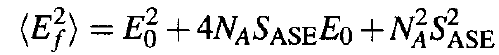The standard deviation of energy fluctuations is given by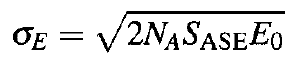As expected on physical grounds, σE scales with the number of amplifiers as, or with the link length as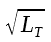since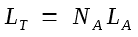for an amplifier spacing of LA. Even for a relatively long fiber link with 100 cascaded amplifiers, the level of energy fluctuations remains below 10% for typical parameter values.

#### 4. Noise-Induced Timing Jitter

Let us now calculate the timing jitter at the end of a fiber link with a cascaded chain of NA amplifiers. Equationcan be integrated in a straightforward manner to obtain the total jitter at the end of a fiber link in the form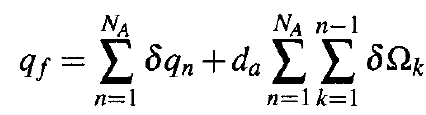whereis the accumulated dispersion in each fiber section with average dispersion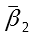. Timing jitter is calculated from this equation using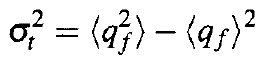where angle brackets denote averaging over the random variables δqk and δΩk. Since both of them vanish on average, it follows that ⟨qf⟩ = 0. However, the variance σ2t remains finite.

The average appearing in the qf equation above can be carried out analytically. Since the whole procedure is well known, we only state the final resultIn the case of perfect dispersion compensation such that da = 0, the jitter variance increases linearly with the number of amplifiers. In contrast, when da ≠ 0, it increases with NA in a cubic fashion. For such long-haul systems, the dominant term in this following equation varies as N3Ad2a.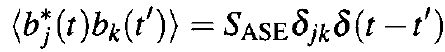This is a general feature of ASE-induced jitter. The cubic dependence of timing jitter on system length shows that even relatively small frequency fluctuations can induce enough jitter in long-haul systems that they may become inoperable, especially when fiber dispersion is not compensated.

Residual dispersion of a fiber link can lead to considerable timing jitter in CRZ systems, as seen from the σ2t equation above. The following figure shows the timing jitter as a function of the total system length LT = NALA for a 10-Gb/s system using four values ofwith Tm = 30 ps, LA = 50 km, C0 = 0.2, and SASE/E0 = 10-4.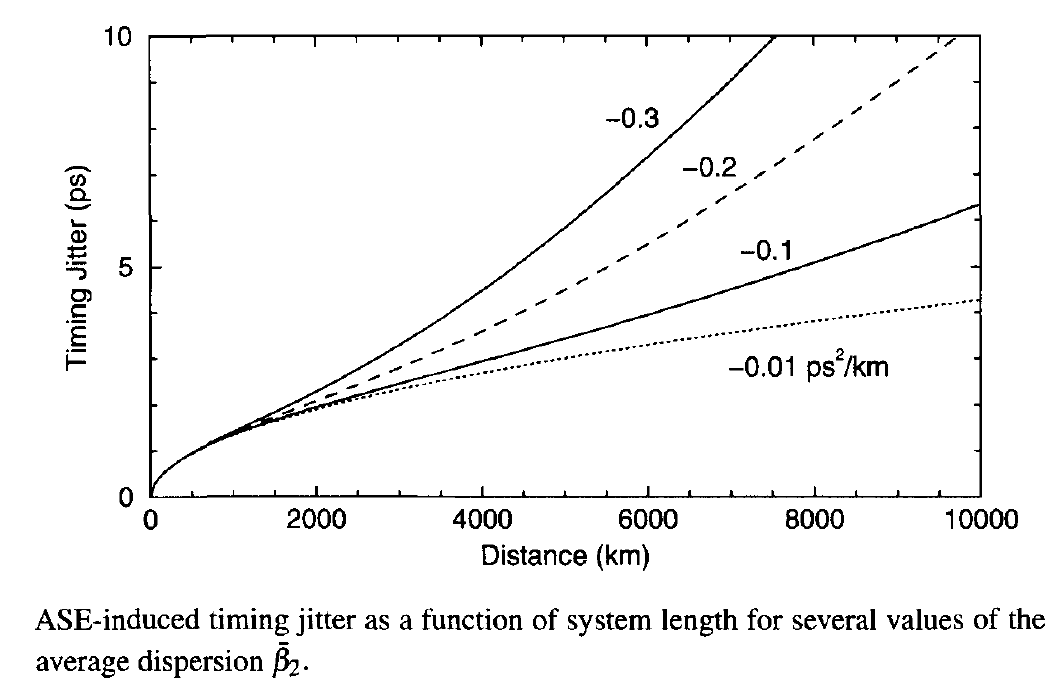The ASE-induced jitter becomes a significant fraction of the pulse width for values ofas small as 0.2 ps2/km because of the cubic dependence of σ2t on the system length LT. Such jitter would lead to large power penalties, if left uncontrolled. The use of distributed amplification lowers the noise level in lightwave systems. One would thus expect timing jitter to be reduced if Raman amplification is employed in place of lumped amplifiers. This indeed turns out to be the case.